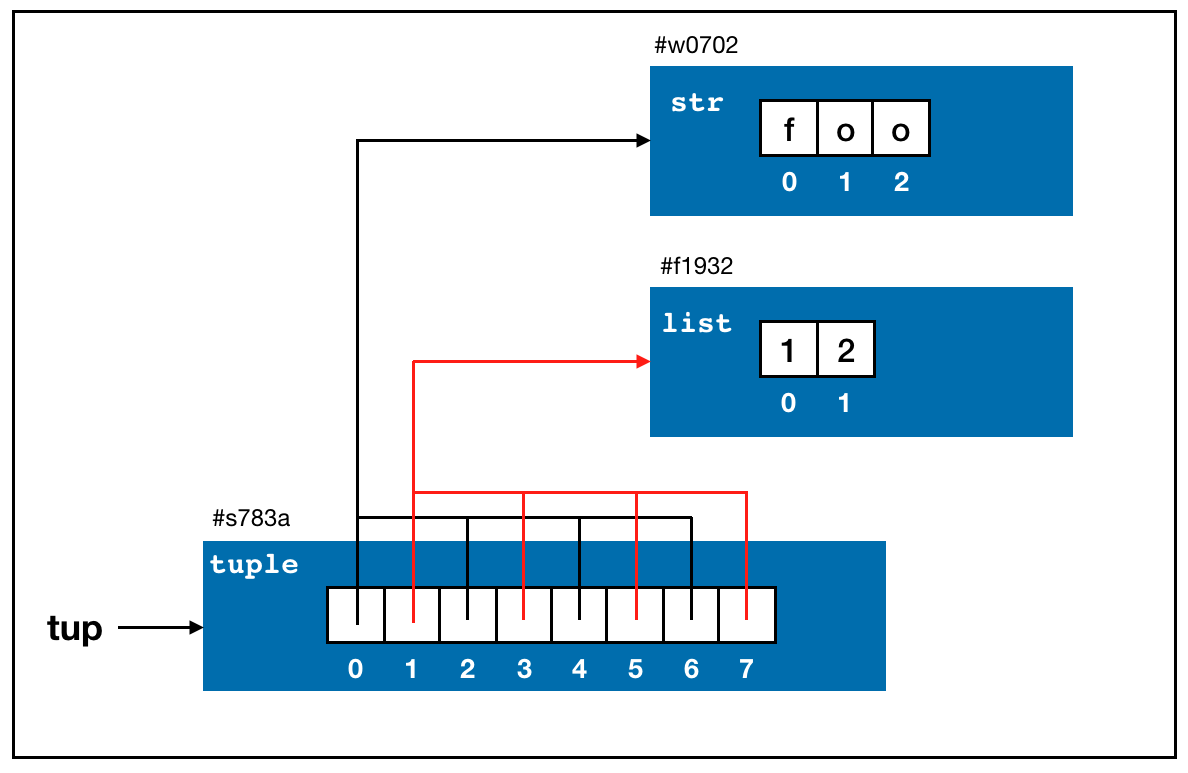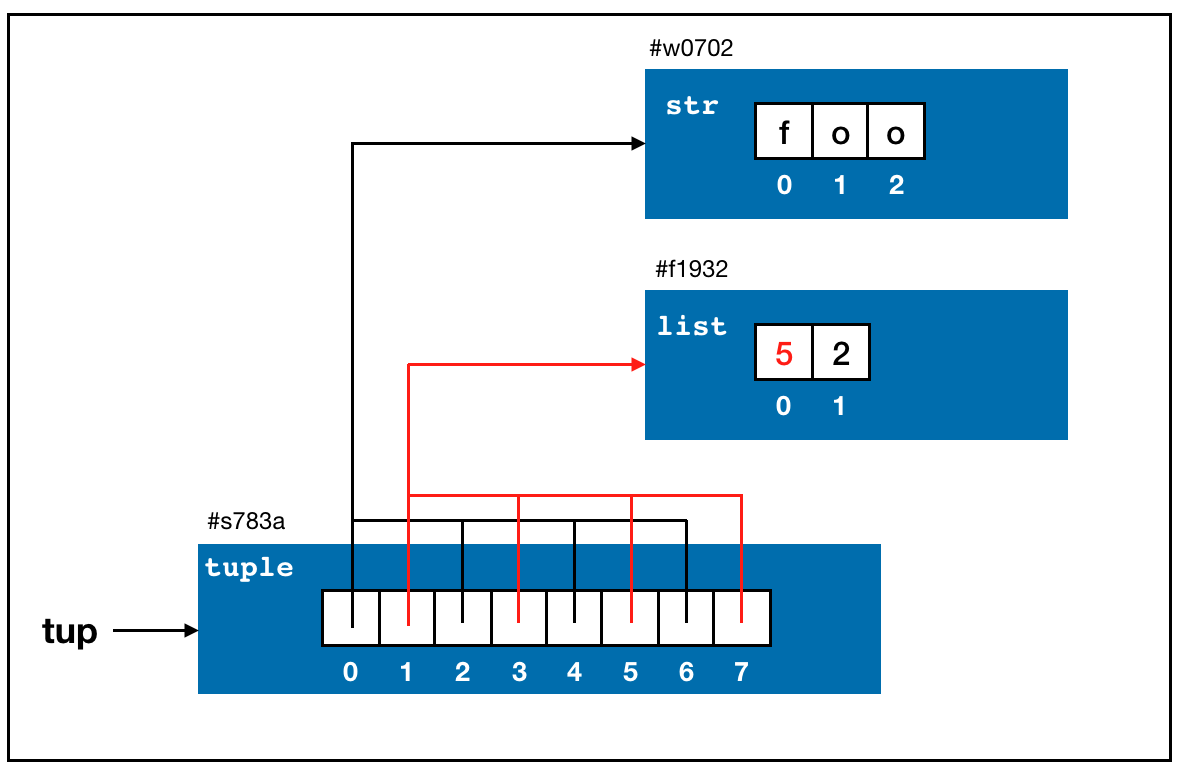## 1. Tuple

• A tuple is a fixed-length, immutable sequence of Python objects.
tup = 4, 5, 6
tup
## (4, 5, 6)
tup2 = (1, 2)
tup2
## (1, 2)
• A tuple can contain objects of different types!
• A big difference between Python tuple and R vector!
tup3 = (1, "2", True)
tup3
## (1, '2', True)
• You can also create a tuple of tuples:
nested_tup = (4, 5, 6), (7, 8)
nested_tup
## ((4, 5, 6), (7, 8))
• You can convert any sequence or iterator to a tuple by invoking tuple():
tuple([4, 0, 2])
## (4, 0, 2)
tuple("string")
## ('s', 't', 'r', 'i', 'n', 'g')

## 2. Elements of a Tuple

• Elements of a tuple can be accessed with square brackets []:
• Note: In Python (unlike R), index starts at 0.
tup = ('hello', 2, 'you')
tup
## 'hello'
• While the objects stored in a tuple may be mutable themselves, once the tuple is created, it’s not possible to modify which object is stored in each slot.
e1 = 'foo'
e2 = [1, 2]
tup = (e1, e2)
tup
## ('foo', [1, 2])
• We know e1 (string) is immutable and e2 (list) is mutable.
e2 = 3
e2
## [3, 2]
tup
## ('foo', [3, 2])
tup = 4
tup
## ('foo', [3, 4])

## 3. Tuple Concatenation

• You can concatenate tuples using the + operator to produce longer tuples:
• Note that this is NOT the same as mutate/change the original tuple!
(4, None, 'foo') + (6, 0) + ('bar',)
## (4, None, 'foo', 6, 0, 'bar')
• Multiplying a tuple by an integer, as with lists, has the effect of concatenating together that many copies of the tuple:
tup = ('foo', [1, 2])*4
tup
## ('foo', [1, 2], 'foo', [1, 2], 'foo', [1, 2], 'foo', [1, 2])• Note that the objects themselves are not copied, only the references to them.
tup = 5
tup
## ('foo', [5, 2], 'foo', [5, 2], 'foo', [5, 2], 'foo', [5, 2])## 4. Unpacking Tuples

• If you try to assign to a tuple-like expression of variables, Python will attempt to unpack the value on the righthand side of the equals sign:
tup = (4, 5, 6)
a, b, c = tup
a
## 4
b
## 5
c
## 6
• Even sequences with nested tuples can be unpacked:
tup = 4, 5, (6, 7)
a, b, (c, d) = tup
d
## 7
• A common use of variable unpacking is iterating over sequences of tuples or lists:
seq = [(1, 2, 3), (4, 5, 6), (7, 8, 9)]
for a, b, c in seq:
print('a={0}, b={1}, c={2}'.format(a, b, c))
## a=1, b=2, c=3
## a=4, b=5, c=6
## a=7, b=8, c=9
• Python also allows unpacking only a few elements at the beginning of a tuple:
values = 1, 2, 3, 4, 5
a, b, *rest = values
a
## 1
b
## 2
rest
## [3, 4, 5]
• The special key here is * (not rest). In fact, you can also run the above code with a, b, *_ = values instead.

## 5. count()

• The count() function/method counts the number of occurrences of a value.
a = (1, 2, 2, 2, 3, 4, 5)
a.count(2)
## 3

## Exercise

Some students’ information is stored in a nested tuple where each inner tuple represents a student.

students = (('Ha', 25, True), ('Alex', 50, False), ('Dave', 34, False), ('Lelys', 50, True))

For each inner tuple, the information is stored in the following format (name, age, is female?).

Unpack this tuple and store all students name in a string (separated by comma ,), and all students’ ages together and count the number of female and male students (store them in female_count and male_count).

This lecture note is modified from Chapter 3 of Wes McKinney’s Python for Data Analysis 2nd Ed.